# figure

Create figure window

## Syntax

``figure``
``figure(Name,Value)``
``f = figure(___)``
``figure(f)``
``figure(n)``

## Description

````figure` creates a new figure window using default property values. The resulting figure is the current figure.```

example

````figure(Name,Value)` modifies properties of the figure using one or more name-value pair arguments. For example, `figure('Color','white')` sets the background color to white.```

example

````f = figure(___)` returns the `Figure` object. Use `f` to query or modify properties of the figure after it is created.```

example

````figure(f)` makes the figure specified by `f` the current figure and displays it on top of all other figures.```
````figure(n)` finds a figure in which the `Number` property is equal to `n`, and makes it the current figure. If no figure exists with that property value, MATLAB® creates a new figure and sets its `Number` property to `n`.```

## Examples

collapse all

Create a default figure.

`f = figure;`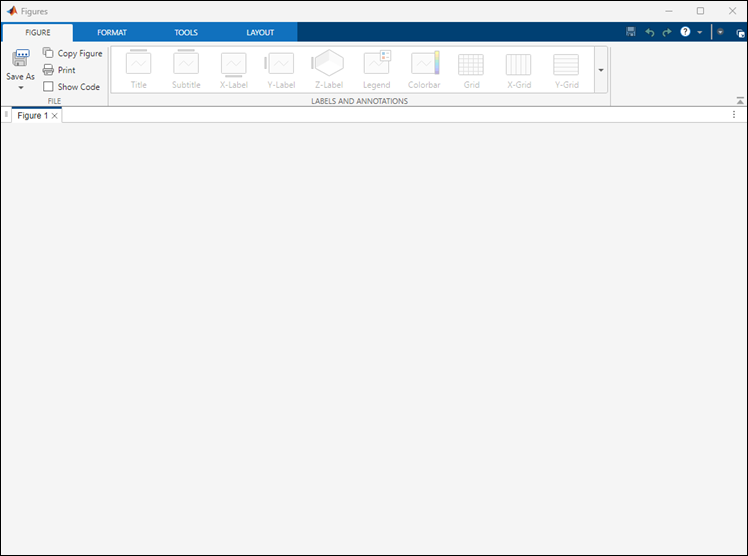Get the location, width, and height of the figure.

`f.Position`
```ans = 680 558 560 420```

This means that the figure window is positioned 680 pixels to the right and 558 pixels above the bottom left corner of the primary display, and is 560 pixels wide and 420 pixels tall.

Halve the figure width and height by adjusting the third and fourth elements of the position vector.

`f.Position(3:4) = [280 210];`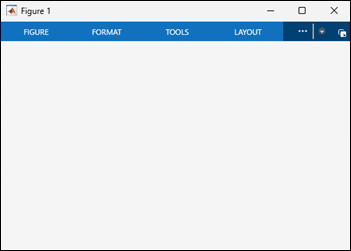Create a figure, and specify the `Name` property. By default, the resulting title includes the figure number.

`figure('Name','Measured Data');`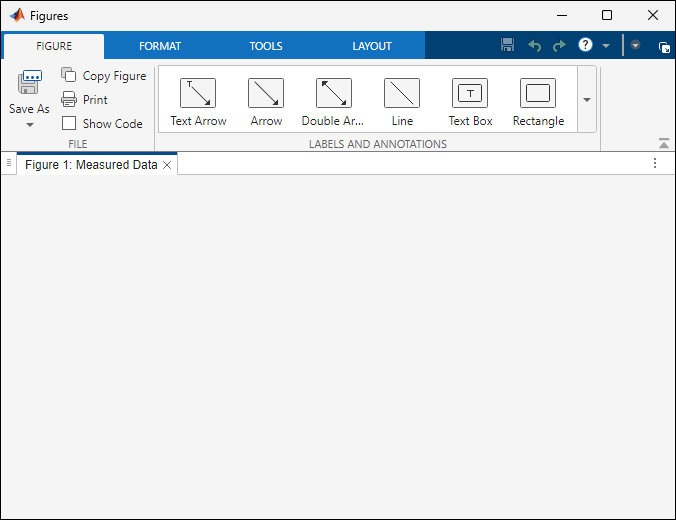Specify the `Name` property again, but this time, set the `NumberTitle` property to `'off'`. The resulting title does not include the figure number.

`figure('Name','Measured Data','NumberTitle','off');`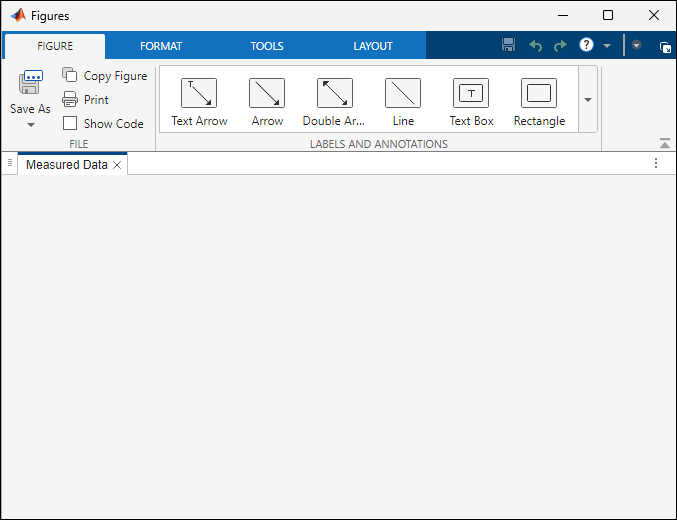Create two figures, and then create a line plot. By default, the `plot` command targets the current figure.

```f1 = figure; f2 = figure; plot([1 2 3],[2 4 6]);```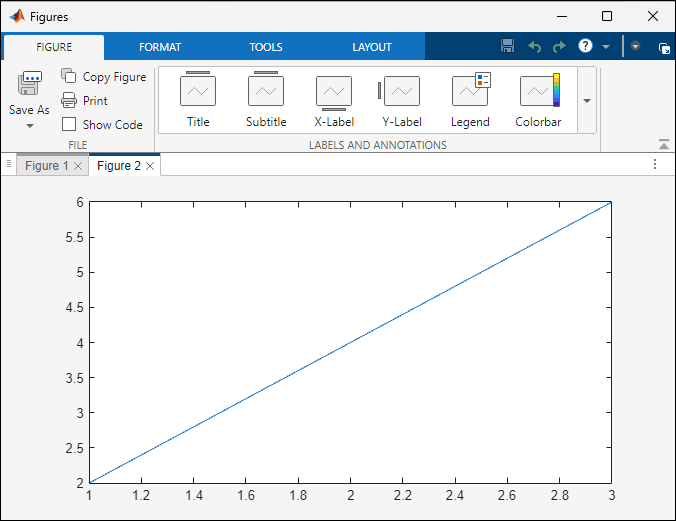Set the current figure to `f1`, so that it is the target for the next plot. Then create a scatter plot.

```figure(f1); scatter((1:20),rand(1,20));```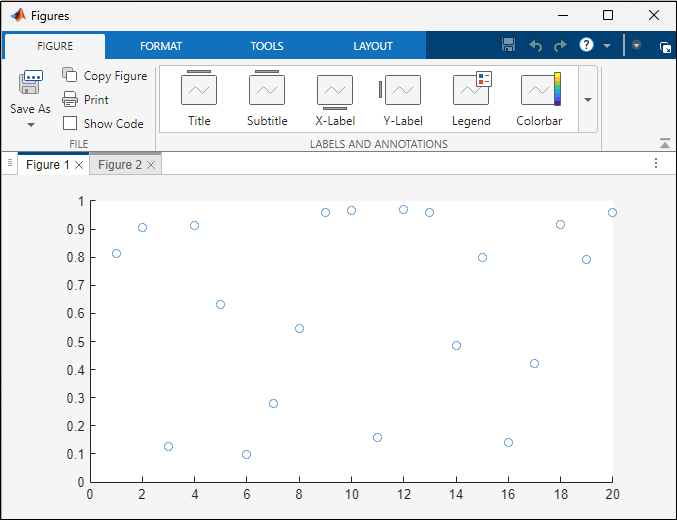## Input Arguments

collapse all

Target figure, specified as a `Figure` object.

Target figure number, specified as a scalar integer value. When you specify this argument, MATLAB searches for an existing figure in which the Number property is equal to `n`. If no figure exists with that property value, MATLAB creates a new figure and sets its `Number` property to `n`. By default, the `Number` property value is displayed in the title of the figure.

Data Types: `double`

### Name-Value Arguments

Example: `figure('Color','white')` creates a figure with a white background.

Specify optional comma-separated pairs of `Name,Value` arguments. `Name` is the argument name and `Value` is the corresponding value. `Name` must appear inside single quotes (`' '`). You can specify several name and value pair arguments as `Name1,Value1,...,NameN,ValueN`.

Note

The properties listed here are only a subset. For a full list, see Figure Properties.

Name of the figure, specified as a character vector or a string scalar.

Example: `figure('Name','Results')` sets the name of the figure to `'Results'`.

By default, the name is `'Figure n'`, where `n` is an integer. When you specify the `Name` property, the title of the figure becomes ```'Figure n: name'```. If you want only the `Name` value to appear, set `IntegerHandle` or `NumberTitle` to `'off'`.

Background color, specified as an RGB triplet, a hexadecimal color code, a color name, or a short name. If you specify `'none'`, the background color appears black on screen, but if you print the figure, the background prints as though the figure window is transparent.

For a custom color, specify an RGB triplet or a hexadecimal color code.

• An RGB triplet is a three-element row vector whose elements specify the intensities of the red, green, and blue components of the color. The intensities must be in the range `[0,1]`; for example, ```[0.4 0.6 0.7]```.

• A hexadecimal color code is a character vector or a string scalar that starts with a hash symbol (`#`) followed by three or six hexadecimal digits, which can range from `0` to `F`. The values are not case sensitive. Thus, the color codes `'#FF8800'`, `'#ff8800'`, `'#F80'`, and `'#f80'` are equivalent.

Alternatively, you can specify some common colors by name. This table lists the named color options, the equivalent RGB triplets, and hexadecimal color codes.

Color NameShort NameRGB TripletHexadecimal Color CodeAppearance
`'red'``'r'``[1 0 0]``'#FF0000'``'green'``'g'``[0 1 0]``'#00FF00'``'blue'``'b'``[0 0 1]``'#0000FF'``'cyan'` `'c'``[0 1 1]``'#00FFFF'``'magenta'``'m'``[1 0 1]``'#FF00FF'``'yellow'``'y'``[1 1 0]``'#FFFF00'``'black'``'k'``[0 0 0]``'#000000'``'white'``'w'``[1 1 1]``'#FFFFFF'``'none'`Not applicableNot applicableNot applicableNo color

Here are the RGB triplets and hexadecimal color codes for the default colors MATLAB uses in many types of plots.

`[0 0.4470 0.7410]``'#0072BD'``[0.8500 0.3250 0.0980]``'#D95319'``[0.9290 0.6940 0.1250]``'#EDB120'``[0.4940 0.1840 0.5560]``'#7E2F8E'``[0.4660 0.6740 0.1880]``'#77AC30'``[0.3010 0.7450 0.9330]``'#4DBEEE'``[0.6350 0.0780 0.1840]``'#A2142F'`Data Types: `double` | `char`

Location and size of the drawable area, specified as a vector of the form `[left bottom width height]`. This area excludes the figure borders, title bar, menu bar, and tool bars.

This table describes each element in the `Position` vector.

ElementDescription
`left`Distance from the left edge of the primary display to the inner left edge of the window. This value can be negative on systems that have more than one monitor.

If the figure is docked, then this value is relative to the Figure panel within the MATLAB desktop.
`bottom`Distance from the bottom edge of the primary display to the inner bottom edge of the window. This value can be negative on systems that have more than one monitor.

If the figure is docked, then this value is relative to the Figure panel within the MATLAB desktop.
`width`Distance between the right and left inner edges of the figure.
`height`Distance between the top and bottom inner edges of the window.

All measurements are in units specified by the `Units` property.

You cannot specify the figure `Position` property when the figure is docked.

In MATLAB Online™, the `bottom` and `left` elements of the `Position` vector are ignored.

To place the full window, including the borders, title bar, menu bar, tool bars, use the `OuterPosition` property.

Note

The Windows® operating system enforces a minimum window width and a maximum window size. If you specify a figure size outside of those limits, the displayed figure will conform to the limits instead of the size you specified.

Units of measurement, specified as one of the values from this table.

Units ValueDescription
`'pixels'` (default)

Pixels.

Starting in R2015b, distances in pixels are independent of your system resolution on Windows and Macintosh systems:

• On Windows systems, a pixel is 1/96th of an inch.

• On Macintosh systems, a pixel is 1/72nd of an inch.

On Linux® systems, the size of a pixel is determined by your system resolution.

`'normalized'`These units are normalized with respect to the parent container. The lower-left corner of the container maps to `(0,0)` and the upper-right corner maps to `(1,1)`.
`'inches'`Inches.
`'centimeters'`Centimeters.
`'points'`Points. One point equals 1/72nd of an inch.
`'characters'`

These units are based on the default uicontrol font of the graphics root object:

• Character width = width of the letter `x`.

• Character height = distance between the baselines of two lines of text.

To access the default uicontrol font, use `get(groot,'defaultuicontrolFontName')` or `set(groot,'defaultuicontrolFontName')`.

MATLAB measures all units from the lower left corner of the parent object.

This property affects the `Position` property. If you change the `Units` property, consider returning its value to the default value after completing your computation to avoid affecting other functions that assume the default value.

The order in which you specify the `Units` and `Position` properties has these effects:

• If you specify the `Units` before the `Position` property, then MATLAB sets `Position` using the units you specify.

• If you specify the `Units` property after the `Position` property, MATLAB sets the position using the default `Units`. Then, MATLAB converts the `Position` value to the equivalent value in the units you specify.

collapse all

### Current Figure

The current figure is the target for graphics commands such as `axes` and `colormap`. Typically, it is the last figure created or the last figure clicked with the mouse. The `gcf` command returns the current figure.

## Tips

• Use the graphics root object to set default values on the root level for other types of objects. For example, set the default colormap for all future figures to the `summer` colormap.

`set(groot,'DefaultFigureColormap',summer)`
To restore a property to its original MATLAB default, use the `'remove'` keyword.
`set(groot,'DefaultFigureColormap','remove')`